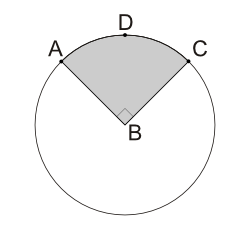We cover every section of the GMAT with in-depth lessons, 5000+ practice questions and realistic practice tests.

## Up to 90+ points GMAT score improvement guarantee

### The best guarantee you’ll find

Our Premium and Ultimate plans guarantee up to 90+ points score increase or your money back.

## Master each section of the test

### Comprehensive GMAT prep

We cover every section of the GMAT with in-depth lessons, 5000+ practice questions and realistic practice tests.

## Schedule-free studying

### Learn on the go

Study whenever and wherever you want with our iOS and Android mobile apps.

# Circles: Method for Solving Questions involving Arcs and AnglesIf $$B$$ is the center of the circle in the figure above and the area of the shaded region is $$16 \pi$$, what is the length of arc $$ADC$$?

Next use this to find the circle's radius: > $$\pi r^2=64 \pi$$ > $$r^2=64$$ > $$r=8$$

Now, find the circle's circumference:

> $$\text{Circumference} = 2\pi r = 2\pi(8) =16 \pi$$ Since Arc $$ADC$$ is one-fourth of the circumference, >$$\text{Arc } ADC = \frac{1}{4} \times 16 \pi = 4 \pi$$.

Incorrect.

[[snippet]]

Incorrect.

[[snippet]]

This is the value of the circle's circumference:

$$2 \pi r = 2\times8\times \pi = 16 \pi$$

Now, you have one more step . . .

Incorrect.

[[snippet]]

Incorrect.

[[snippet]]
Correct. [[snippet]] When you plug in the given information, you get > $$\displaystyle{\frac{16 \pi}{\text{Circle Area}}=\frac{90^\circ}{360^\circ}}$$ > $$\displaystyle{\frac{16 \pi}{\text{Circle Area}}=\frac{1}{4}}$$ Therefore, > $$\text{Circle Area} = 4\times16 \pi = 64 \pi$$
$$4$$
$$4 \pi$$
$$16$$
$$8 \pi$$
$$16 \pi$$
Continue